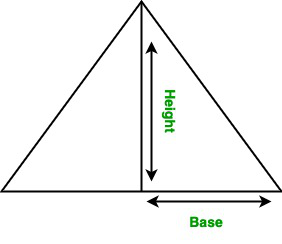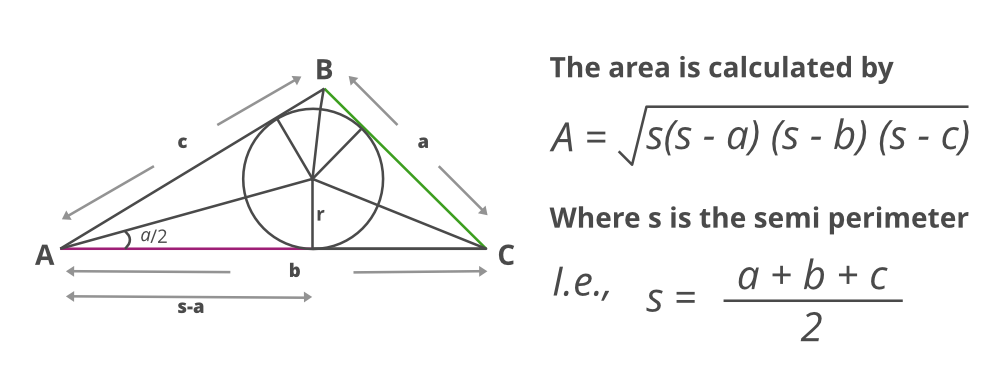Open in App
Not now

# Java Program to Find the Area of a Triangle

• Last Updated : 19 Jul, 2022

A triangle is a polygon. It has three edges and three vertices and each vertex from an angle. It is a closed 2-dimensional shape. In this article, we will learn how to find the area of the triangle.

There can be two possibilities while calculating the area of the triangle as per cases

• Using the height and base of the triangle
• Using the 3 sides of the triangle

Case 1: When the height and base of the triangle are given, then the area of the triangle is half the product of its base and height.Formula:

Area of triangle: Area = (height×base)/2

Example 1: Evaluation of area using base and height

## Java

 `// Java program to find the``// area of the triangle` `// Importing java libraries``import` `java.io.*;` `class` `GFG {` `    ``// Function to calculate the``    ``// area of the triangle``    ``static` `double` `area(``double` `h, ``double` `b)``    ``{``        ``// Function returning the value that is``        ``// area of a triangle``        ``return` `(h * b) / ``2``;``    ``}` `    ``// Main driver code``    ``public` `static` `void` `main(String[] args)``    ``{``        ``// Custom inputs- height and base values` `        ``// Height of the triangle``        ``double` `h = ``10``;` `        ``// Base of the triangle``        ``double` `b = ``5``;` `        ``// Calling area function and``        ``// printing value corresponding area``        ``System.out.println(``"Area of the triangle: "``                           ``+ area(h, b));``    ``}``}`

Output:

`Area of the triangle: 25.0`

Time complexity: O(1)

Auxiliary Space: O(1)

Case 2: When the three sides of the triangle are given

Now suppose if only sides are known to us then the above formula can not be applied. The area will be calculated using the dimensions of a triangle. This formula is popularly known as Heron’s formula.Algorithm:

1. The Semiperimeter of the triangle is calculated.
2. Product of semi meter with 3 values where these rest of values are the difference of sides from above semi perimeter calculated.
3. Square rooting the above value obtained from the computations gives the area of a triangle.

Example 2:

## Java

 `// Java program to find the area of``// the triangle using Heron’s formula` `// Importing java libraries``import` `java.io.*;` `class` `GFG {` `    ``// Function to calculate the area where parameters``    ``// passed are three sides of a triangle``    ``static` `float` `area(``float` `r, ``float` `s, ``float` `t)``    ``{``        ``// Condition check over sides of triangle``        ``if` `(r < ``0` `|| s < ``0` `|| t < ``0` `|| (r + s <= t)``            ``|| r + t <= s || s + t <= r)` `        ``// Length of sides must be positive and sum of``        ``// any two sides must be smaller than third side` `        ``{``            ``// print message if condition fails``            ``System.out.println(``"Not a valid input"``);``            ``System.exit(``0``);``        ``}` `        ``/*else*/` `        ``// Finding Semi perimeter of the triangle``        ``// using formula``        ``float` `S = (r + s + t) / ``2``;` `        ``// Finding the area of the triangle``        ``float` `A = (``float``)Math.sqrt(S * (S - r) * (S - s)``                                   ``* (S - t));` `        ``// return area value``        ``return` `A;``    ``}` `    ``// Main driver code``    ``public` `static` `void` `main(String[] args)``    ``{``        ``// custom inputs of sides of values` `        ``// Sides of the triangle``        ``float` `r = ``5``.0f;``        ``float` `s = ``6``.0f;``        ``float` `t = ``7``.0f;` `        ``// Calling area function and``        ``// printing the area of triangle``        ``System.out.println(``"Area of the triangle: "``                           ``+ area(r, s, t));``    ``}``}`

Output:

`Area of the triangle: 14.6969385`

Time complexity: O(logn)

Auxiliary Space: O(1)

My Personal Notes arrow_drop_up